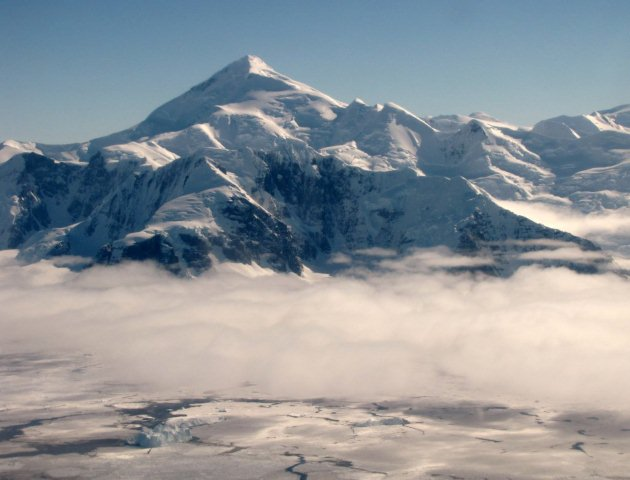# Calculating glacier ice volumes and sea level equivalents

## How do we estimate the global volume of ice?

### Sea level equivalents

The IPCC and other outlets frequently indicate how much sea levels will rise under given climate change scenarios (mm sea level rise) . Other times, you might see that such and such volume of ice (km3) is equivalent to so many millimetres of sea level rise (sea level equivalent (SLE); the amount of sea level rise on full melting of the ice). But how are these calculations made?

Table 1. Sea level equivalent (SLE) from various land ice sources. From Morligheim et al. (2017; 2019) and Farinotti et al. 2019.

### Antarctica

The first complexity is in calculating the volume of ice in the world. This is complex, as we often do not have a complete picture of the bed of the ice sheet or glacier. For Antarctica, BEDMAP2 and Bedmachine provides the most complete and up-to-date estimate of ice volume, and it is derived by combining thousands of radar and seismic measurements of ice thickness [2,3].

In fact, BEDMAP 2 is derived from 25 million measurements. Fretwell et al. 2013 estimated that the Antarctic Ice Sheet comprised 27 million km3 of ice, with a sea level equivalent of ~58 m. BedMachine estimates the sea level equivalent of Antarctica to be 57.9 ± 0.9 m .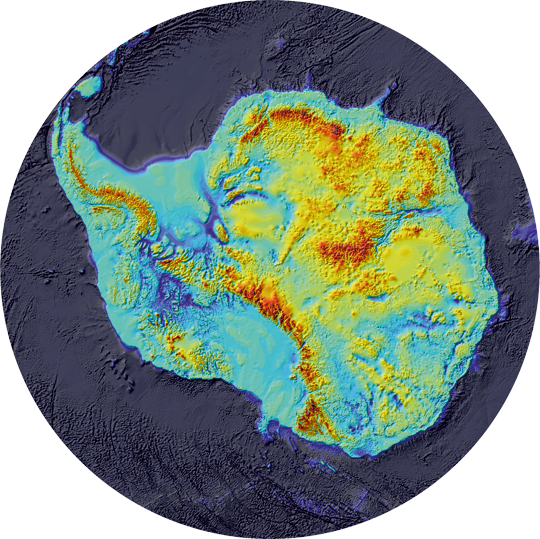BEDMAP 2, showing that the bedrock on which the West Antarctic Ice Sheet rests is well below sea level. The Antarctic Ice Sheet has a sea level equivalent of 58.3 m (i.e., if all the ice in Antarctica melted, this is how much global sea levels would rise).

### Greenland

BedMachine V3 provides a recent estimate of ice volume for the Greenland Ice Sheet .

In Greenland, the bed topography is a primary control on ice flow, grounding line migration and calving, and subglacial drainage. Deep fjords allow penetration of warm Atlantic Water that undercuts Greenland’s tidewater glaciers that terminate in the ocean. This means that sectors of the Greeland Ice Sheet, like the Antarctic Ice Sheet, are also vulnerable to oceanic forcing.

The BedMachine V3 is a 150 m horizontal resolution bed topography and bathymetric map, which shows that the sea level potential of the Greenland Ice Sheet is 7.42 ± 0.05 m .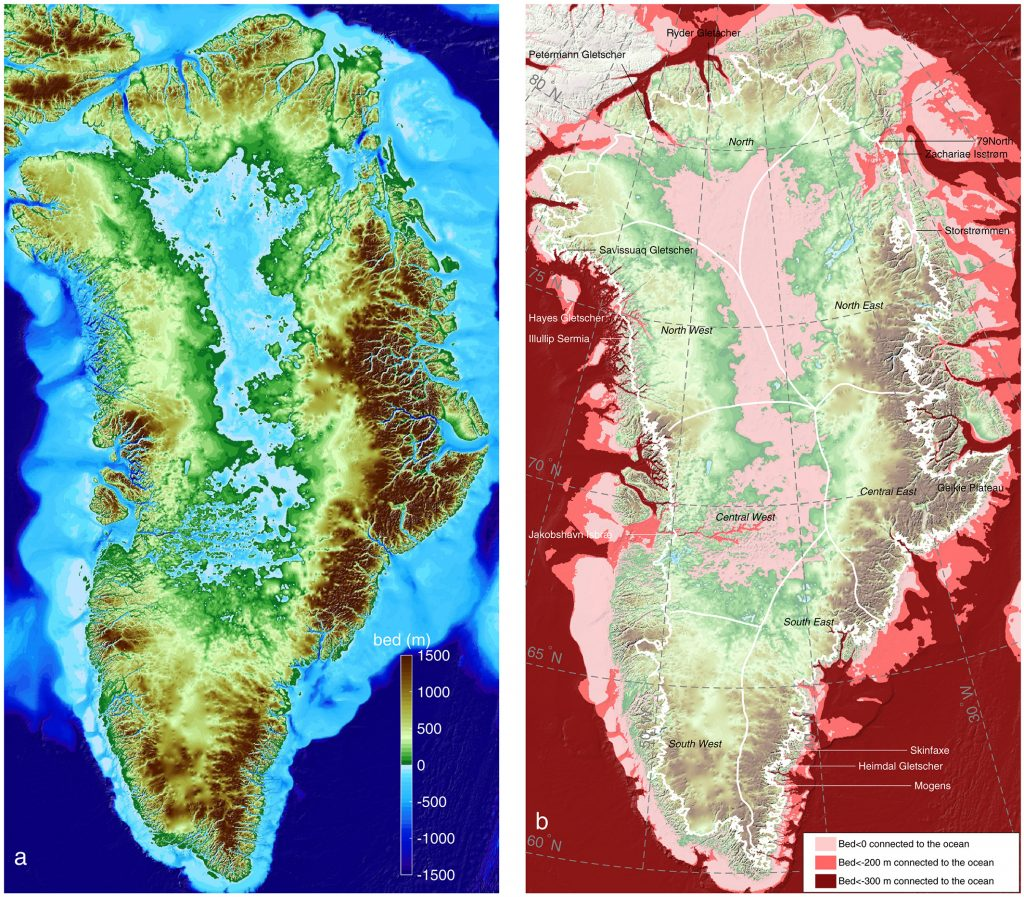(a) BedMachine V3 bed topography, with areas below sea level in blue. (b) regions below sea level (light pink) that are connected to the ocean and maintain a depth below 200 m (dark pink). Morligheim et al., 2017

### Global glaciers

For glaciers, ice thickness datasets are sparse .  While we have a good estimate of global glacier ice surface area  from satellite measurements, direct observations of glacier ice thickness are available for only around 3000 glaciers [5, 7, 8; Welty et al.]. These include radar measurements (both airbourne and from the ice surface) and seismic measurements. Unfortunately, these methods are time consuming and costly, and glaciers are often remote and difficult to access.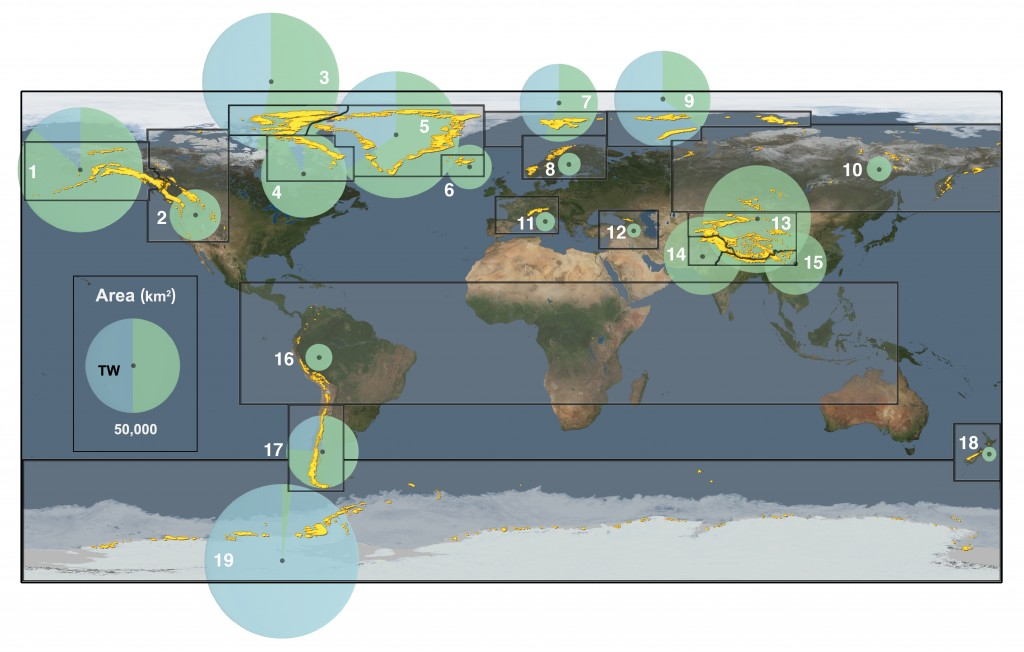This figure shows the global distribution of glaciers. The diameter of the circle shows the area covered. The area covered by tidewater glaciers is shown in blue. The number refers to the RGI region. From the IPCC AR5 Working Group 113. The sea level equivalent of glaciers is important because glaciers are responding rapidly to climate change and are likely to be a large contributor to global sea level rise over the next century.

Yet glacier volume and ice thickness data are essential parameters required for understanding sea level rise, water resource management and assessment of future glacier changes . Glaciers and small ice caps are also contributing rapidly to present-day sea level rise [9, 10]. Ice thickness data and bed topography are essential data required for numerical modelling of glaciers .

Other methods employed to calculate glacier volume include volume-area scaling [12-16], and calculations of ice thickness based on ice surface slope [16, 17] that assume a given basal shear stress, and numerical models based on principles of ice physics and mass conservation . These methods, when correctly applied, do well against direct observations of ice thickness [11, 13, 18].

Farinotti et al. (2019) provided an updated estimate for ice thickness for all glaciers on Earth. They used an ensemble of five models to provide a consensus esimate for 215,000 glaciers outside of the Greenland and Antarctic ice sheets. They find a total volume of 158 ± 41 x103 km3.

Taking into account and discounting the ice below present-day sea level, this is equivalent to 0.32 ± 0.08 m of sea-level equivalent . Roughly 15% of glacier ice is below sea level.

These data have been compiled and can be explored in the “World Glacier Explorer” app in OGGM-Edu.

## Calculating the mass of ice

### Gigatonnes

The mass of ice is usually given in metric gigatonnes (Gt). 1 Gt = 109 tonnes (where 1 tonne = 1000 kg); a gigatonne is 1 billion tonnes.

A tonne of water occupies one cubic metre (a cube 1m x 1 m x 1m). A gigatonne (Gt) occupies one cubic kilometre of water (1km x 1km x 1km).

### Densities of ice and water

Calculating the sea level equivalent for a given volume of ice requires some simple maths and a knowledge of the densities and properties of ice and sea water.  Ice volumes are usually given in km3.

Table 2. Densities of ice and water (at 1 atmospheric pressure and 4.3°C)

We can calculate the mass of something if we know the volume and the density:

Density = Mass / Volume

Mass = Volume x Density

Volume = Mass / Density

See this website for more information on these equations.

Because ice and water are different densities, 1 km3 results in different masses. However, remember that 1 Gt of ice = 1 Gt of water! They take up different volumes but have the same mass. So, 1 Gt (whether ice or water) is equal to:

• 1.091 km3 ice
• 1.000 km3 pure water
• 0.9737 km3 sea water

### Converting a volume (km3 ) to a mass of ice (Gt)

We can convert a given volume of ice (in km3) to a mass of ice (in Gt) by using the following equation:

Mass of ice (Gt) = Volume of ice (km3) x Density of ice (Gt/km3)

If we have a calculated ice volume of 500 km3, then:

Mass of ice = 500 x 0.9167

The mass of ice here is 458.30 Gt. If it all melted, you would have 458.30 Gt of water.

## Calculating sea level equivalent

### Removing ice below sea level and floating ice

Calculating the sea level equivalent from a mass of ice involves firstly, removing all ice below sea level. This ice is already displacing water, and will not raise sea levels upon melting. This is explained in excellent detail here.

Floating ice (ice shelves, sea ice, floating ice tongues) are already floating and displacing water, and so these ice masses do not raise sea levels upon melting.

However, increased ice discharge, which delivers more icebergs to the ocean, will raise sea levels as ice that was not floating is delivered into the ocean.

The ice that is excluded from sea-level equivalent calculations is shown in the figure below. Firstly, the ice volume, ice thickness and bed topogrpahy must be known. Then, ice below sea level is excluded (it already displaces water) (2).

In (3) below, ice that is below hydrostatic equilibrium is excluded as it will not contribute to sea level rise (only ice that is above freeboard will contribute to sea level rise).

Finally, in (4) a firn air correction is applied; the snow and firn near the upper surface includes a lot of air. If you remove all the air, the surface of the ice sheet would be slightly lower.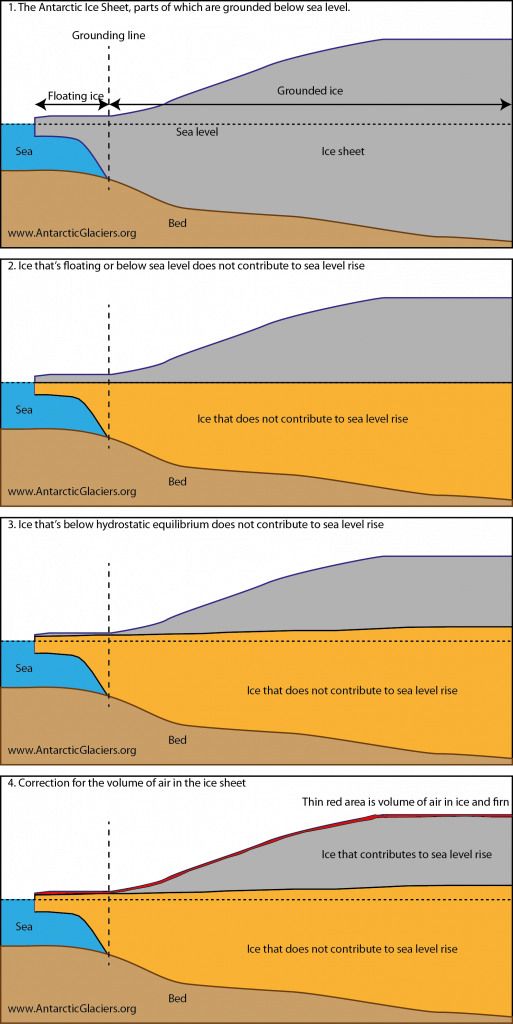Calculating the amount of ice that contributes to sea level rise. Only ice above hydrostatic equilibrium can contribute to sea level rise. Ice below sea level and ice that is floating is excluded from sea level equivalent calculations. The amount of air in firn and ice is also excluded.

### Converting ice volume to sea level rise

To convert a mass of ice into the total amount global sea levels would rise if the ice all melted (i.e., the sea level equivalent), we need to know how much area the oceans cover. This is usually given as 3.618 x 108 km2. A 1 mm increase in global sea level requires 10-3 m3 (10-12 km3) of water for each square metre of the ocean surface, or 10-12 Gt of water.

We can calculate the volume of water required to raise global sea levels by 1 mm:

Volume = area x height

Area = 3.618 x 108 km2

Height = 10-6 km (1 mm)

Volume (km3) = (3.618 x 108 km2 ) x (10-6 km) = 3.618 x 102 km3 = 361.8 kmwater.

We can convert km3 of water to Gt of water as we did above; 1 kmwater = 1 Gt water.  In the same way, 1 Gt of ice = 1 kmwater. So, 361.8 Gt of ice will raise global sea levels by 1 mm. 361.8 Gt of ice is equivalent to 394.67 km3 ice.

### Calculating sea-level equivalent

If we took our 458.30 Gt of ice (as calculated above), then we could calculate the global sea level equivalent by:

SLE (mm) = mass of ice (Gt) x (1 / 361.8)

SLE = 458.30 x (1 / 361.8)

SLE = 1.27 mm

However, we should note that some of the world’s glaciers have parts that are below sea level. This ice will not affect sea level if it melted. The volume of glacier ice below the surface of the ocean should therefore be subtracted from the total volume of glaciers and ice caps when calculating sea level equivalents .

Also remember that ice that is floating (like ice shelves, sea ice and icebergs) does not contribute to sea level rise upon melting. Only land ice above sea level will contribute to sea level rise.

## Useful websites

1. Vaughan, D.G., et al., Observations: cryosphere. Climate change, 2013: p. 317-382.
2. Fretwell, L.O., et al., Bedmap2: improved ice bed, surface and thickness datasets for Antarctica. The Cryosphere, 2013. 7: p. 375-393.
3. Morlighem, M., Rignot, E., Binder, T., Blankenship, D., Drews, R., Eagles, G., … Young, D. A. (2019). Deep glacial troughs and stabilizing ridges unveiled beneath the margins of the Antarctic ice sheet. Nature Geoscience. https://doi.org/10.1038/s41561-019-0510-8
4. Morlighem, M., Williams, C. N., Rignot, E., An, L., Arndt, J. E., Bamber, J. L., … Zinglersen, K. B. (2017). BedMachine v3: Complete Bed Topography and Ocean Bathymetry Mapping of Greenland From Multibeam Echo Sounding Combined With Mass Conservation. Geophysical Research Letters, 44(21), 11,11-51,61. https://doi.org/10.1002/2017GL074954
5. Gärtner-Roer, I., et al., A database of worldwide glacier thickness observations. Global and Planetary Change, 2014. 122(0): p. 330-344.
6. Pfeffer, W.T., et al., The Randolph Glacier Inventory: a globally complete inventory of glaciers. Journal of Glaciology, 2014. 60(221): p. 537.
7. Welty, E., Zemp, M., Navarro, F., Huss, M., Fürst, J. J., Gärtner-Roer, I., … Li, H. (2020). Worldwide version-controlled database of glacier thickness observations. Earth Syst. Sci. Data Discuss., 2020, 1–27. https://doi.org/10.5194/essd-2020-87
8. Andreassen, L., et al., Ice thickness measurements and volume estimates for glaciers in Norway. Journal of Glaciology, 2015. 61(228): p. 763-775.
9. Hock, R., et al., Mountain glaciers and ice caps around Antarctica make a large sea-level rise contribution. Geophysical Research Letters, 2009. 36: p. L07501.
10. Radic, V. and R. Hock, Regionally differentiated contribution of mountain glaciers and ice caps to future sea-level rise. Nature Geosci, 2011. 4(2): p. 91-94.
11. Grinsted, A., An estimate of global glacier volume. The Cryosphere, 2013. 7(1): p. 141-151.
12. Bahr, D.B., Estimation of glacier volume and volume change by scaling methods, in Encyclopedia of Snow, Ice and Glaciers. 2014, Springer. p. 278-280.
13. Bahr, D.B., W.T. Pfeffer, and G. Kaser, A Review of Volume‐Area Scaling of Glaciers. Reviews of Geophysics, 2014.
14. Bahr, D.B., W.T. Pfeffer, and G. Kaser, Glacier volume estimation as an ill-posed inversion. Journal of Glaciology, 2014. 60(223): p. 922-934.
15. Radić, V. and R. Hock, Regional and global volumes of glaciers derived from statistical upscaling of glacier inventory data. Journal of Geophysical Research: Earth Surface, 2010. 115(F1): p. F01010.
16. Marshall, S.J., et al., Glacier Water Resources on the Eastern Slopes of the Canadian Rocky Mountains. Canadian Water Resources Journal / Revue canadienne des ressources hydriques, 2011. 36(2): p. 109-134.
17. Cook, A.J., et al., Glacier retreat on South Georgia and implications for the spread of rats. Antarctic Science, 2010. 22(03): p. 255-263.
18. Farinotti, D., Brinkerhoff, D. J., Clarke, G. K. C., Fürst, J. J., Frey, H., Gantayat, P., … Andreassen, L. M. (2017). How accurate are estimates of glacier ice thickness? Results from ITMIX, the Ice Thickness Models Intercomparison eXperiment. The Cryosphere, 11(2), 949–970. https://doi.org/10.5194/tc-11-949-2017
19. Farinotti, D., Huss, M., Fürst, J. J., Landmann, J., Machguth, H., Maussion, F., & Pandit, A. (2019). A consensus estimate for the ice thickness distribution of all glaciers on Earth. Nature Geoscience. https://doi.org/10.1038/s41561-019-0300-3
20. Haeberli, W. and A. Linsbauer, Brief communication” Global glacier volumes and sea level–small but systematic effects of ice below the surface of the ocean and of new local lakes on land”. The Cryosphere, 2013. 7(3): p. 817-821.

### About Bethan Davies

I am a Senior Lecturer at Newcastle Univeristy, specialising in glaciology and glacial geology. I wrote and developed the AntarcticGlaciers.org website as part of an ongoing commitment to outreach, education and research impact. Read more about me at www.antarcticglaciers.org/bethan-davies.